Georgescu, S., Varodi, A. M., Racasan, S., and Bedelean, B. (2019). "Effect of the dowel length, dowel diameter, and adhesive consumption on bending moment capacity of heat-treated wood dowel joints," BioRes. 14(3), 6619-6632.

#### Abstract

This study applied response surface methodology for modeling and optimizing heat-treated wood dowel joints, the most used joint in furniture construction. The factors examined were dowel length, dowel diameter, and adhesive consumption. The bending moment capacity of the joints loaded in compression or tension were the responses. The load was applied at a constant speed until a major separation between the two parts occurred. To figure out the bending moment capacity, the ultimate failure loads and the moment arms were obtained during testing the joints. The joints were tested by using a universal testing machine. A two-factor interaction model was established to describe the relationship between the factors and the responses. An analysis of variance was employed to test the significance of the developed mathematical model. The dowel length, dowel diameter, and adhesive consumption had significant effects on the bending moment capacity of the heat-treated dowel joints. The dowel length was the main factor that affected the bending moment capacity of the heat-treated dowel joints.

Effect of the Dowel Length, Dowel Diameter, and Adhesive Consumption on Bending Moment Capacity of Heat-treated Wood Dowel Joints

Sergiu Georgescu, Anca Maria Varodi, Sergiu Răcășan, and Bogdan Bedelean *

This study applied response surface methodology for modeling and optimizing heat-treated wood dowel joints, the most used joint in furniture construction. The factors examined were dowel length, dowel diameter, and adhesive consumption. The bending moment capacity of the joints loaded in compression or tension were the responses. The load was applied at a constant speed until a major separation between the two parts occurred. To figure out the bending moment capacity, the ultimate failure loads and the moment arms were obtained during testing the joints. The joints were tested by using a universal testing machine. A two-factor interaction model was established to describe the relationship between the factors and the responses. An analysis of variance was employed to test the significance of the developed mathematical model. The dowel length, dowel diameter, and adhesive consumption had significant effects on the bending moment capacity of the heat-treated dowel joints. The dowel length was the main factor that affected the bending moment capacity of the heat-treated dowel joints.

Keywords: Heat-treated wood; Wood joints; Tension and compression loading; Bending moment capacity Response surface methodology; Optimization

Contact information: Transilvania University of Brasov, Faculty of Wood Engineering, Universității Str. 1, 500036 Brasov, Romania; *Corresponding author: bedelean@unitbv.ro

INTRODUCTION

Heat treatment is an effective method to enhance wood dimensional stability. It has been developed to enhance wood properties and make wood competitive against other materials (Dilik and Hiziroglu 2012; Sandberg et al. 2013). Outdoor furniture is one potential application of heat-treated wood (Sandberg et al. 2013; Kuzman et al. 2015).

The furniture structural system can be classified as frame- or case-type construction (Smardzewski 2015). When a combination of these solutions are observed in the same product, the designed furniture has a composite structure (Eckelman 2003). However, the designed structure must resist to the loads that are going to act on the furniture.

The most used joint in furniture construction is the dowel joint (Fig. 1) because it has favorable cost and production characteristics (Eckelman 2003; Diler et al. 2017). Various factors can affect the strength of dowel joints, including wood species, dowel length, depth of dowel embedment, dowel type, hole diameter, distance between holes, number of dowels, adhesive type and consumption, tightness of fit, boring speed, and feed rate (Eckelman 2003).

Dowel joint sizing (e.g., dowel length, dowel diameter, and adhesive consumption) is based on the studies that have been developed for untreated wood (Curtu et al. 1988; Eckelman 2003; Cismaru 2009; Smardzewski 2015). Because heat-treated wood has inferior mechanical properties compared to untreated wood, an optimization tool could be used in the designing phase of the furniture for appropriate sizing of joints made of heat-treated wood (Tankut et al. 2014).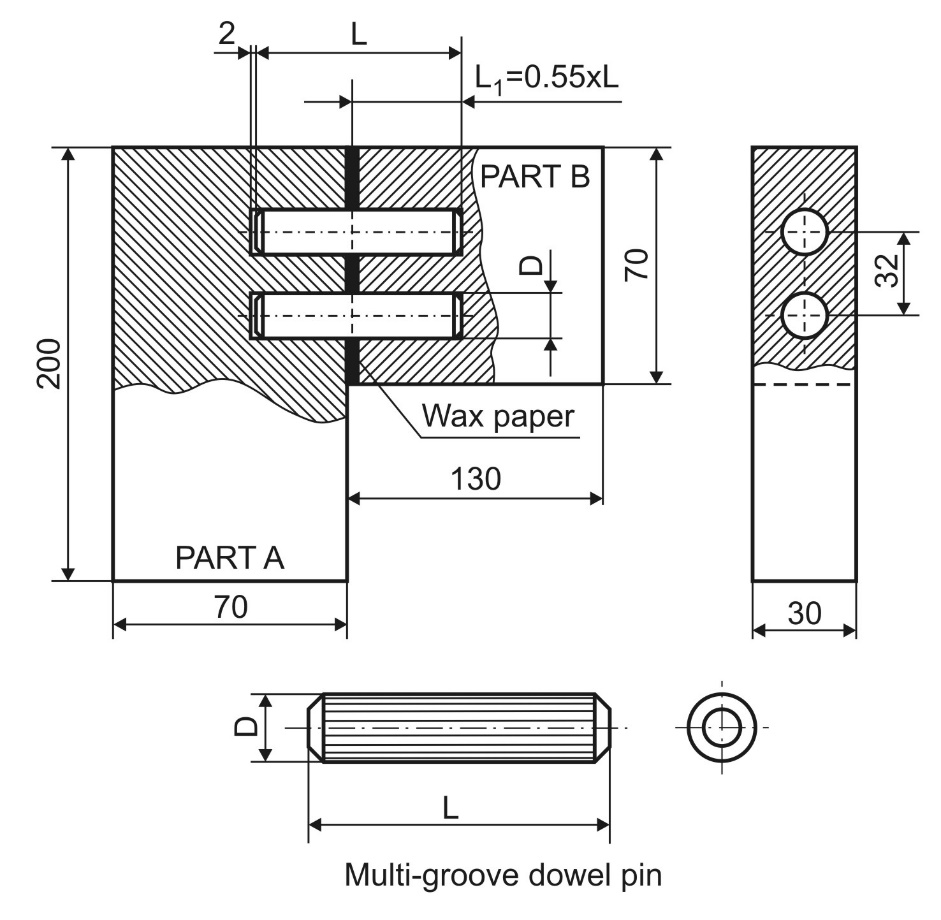Fig. 1. The aspect and main characteristics of the analyzed L-shaped dowel joint (dimensions in mm) (Craftsmanspace 2019)

Moreover, there is limited information on the influence of various factors on the strength of heat-treated wood dowel joints. Kuzman et al. (2015) studied the effect of heat treatment on the mechanical properties of dowel joints produced from untreated and heat-treated beech (Fagus sylvatica L.) and spruce (Picea abies L.). Diler et al. (2017) determined that wood species, heat treatment, adhesive, and joint type have significant effects on the withdrawal force capacity of dowel joints. Georgescu and Bedelean (2017) analyzed the effect of heat treatment, dowel length, dowel spacing, and depth of dowel embedment on the mechanical strength of dowel joints made of ash (Fraxinus excelsior). Their results matched Kuzman et al. (2015): The joints of heat-treated wood had lower compressive and tensile failure loads than the joints of untreated wood. Additionally, Georgescu and Bedelean (2017) reported that the compressive and tensile strengths of heat-treated dowel joints increases when the dowel length increases, the distance between holes increases, and the ratio of dowel embedment in the rail of the joint decreases. However, the analyzed factors could not fully describe the behavior of heat-treated wood dowel joints, and other factors must be considered.

Therefore, the objective of this study was to examine the influence of dowel diameter, adhesive consumption, and dowel length on the bending moment capacity of heat-treated wood dowel joints using response surface methodology (RSM). Design and analysis with RSM consist of designing and executing an experiment to generate response data, fitting the data to a series of polynomial models, conducting an analysis of variance to assess factors’ statistical significance, comparing models, selecting the simplest model that best predicts the analyzed response, and using the selected model to determine the influence of the factors and to reveal the optimal configuration (Anderson and Whitcomb 2005; Yuan et al. 2015).

EXPERIMENTAL

Materials

The wood used in this study, heat-treated ash (Fraxinus excelsior) boards, was obtained from a local sawmill located in Brasov, Romania. Some basic technological steps (namely, straightening, planing, ripping, and crosscutting at final dimensions) were followed to obtain the parts of the dowel joint (parts A (200 x 70 x 30 mm) and B (130 x 70 x 30 mm)) (Fig. 1). Prior to boring, the parts were visually sorted based on several criteria, namely, lack of structural and technological defects and presence of radial annual ring orientation, to maximize the joint reliability (Záborský et al. 2017).

The sorted parts (682 pieces for part A and 703 pieces for part B) were weighed using a digital scale and grouped (based on their weight) into classes using frequency analysis techniques. Parts of the joints were randomly selected from each class, to assure that all groups were similar in terms of material characteristics.

Polyvinyl acetate adhesive (Kleiberit 303 (D4); Kleiberit, Weingarten, Germany) was uniformly dosed and applied in each hole of the joints using a syringe and a glass rod. The quantity of adhesive was calculated by multiplying the adhesive consumption rate by the area of each hole. The possible influence of excess adhesive on the strength of the joints was limited using wax paper (Fig. 1) to separate the parts of the joints (Dalvand et al. 2014).

Multi-grove dowel pins (Fig. 1) made of beech wood (Fagus sylvatica) was used to assemble the parts of the joints. The depth of dowel penetrations in the rail (part B of the joint) was 0.55 from its length (Fig. 1).

The steps to assemble one joint were: applying the adhesive on each wall of the hole; inserting the dowels in the rail; applying the wax paper; mounting the other part of joint; and pressing the joint in a wood clamp until 24 h.

Methods

To obtain the configurations of dowel joints, a central composite design (CCD) was generated with the statistical package Design-Expert® (version 9, Stat-Ease Inc., Minneapolis, MN, USA). In this experimental design, the factors were dowel length (X1), dowel diameter (X2), and adhesive consumption (X3). The responses were bending moment capacities of joints loaded in compression (ŶMC) or tension (ŶMT). The CCD was built up from the following (Anderson and Whitcomb 2005; Ariaee et al. 2014):

• Two-level factorial design points (Fig. 2b) (estimating first-order and two-factor interactions). These points take into account all possible combination of the low (-1) and high (+1) levels of analyzed factors. In this study, there were three factors and each analyzed at two levels (-1, +1). Therefore, there were eight possible combinations (+1, +1, +1), (+1, +1, -1), (+1, -1, -1), (-1,-1,-1), (-1, +1,+1), (-1, -1, +1), (-1, +1, -1) and (+1, -1, +1). Based on these combinations, the configurations #1, 2, 4, 5, 6,11, 12 and 14 of the joints were constructed (Table 1);
• Axial points (estimating pure quadratic effects). Two factors were analyzed at the center value (0) and the third one has the value of alpha (α) (Fig. 2c). Therefore, in this study, there were six possible combinations, namely, (-α, 0, 0), (+α, 0, 0), (0, 0, + α), (0, 0, – α), (0, + α, 0) and (0, – α, 0). Based on these combinations the configurations #3, 7, 8, 9, 10 and 15 of the joints were constructed (Table 1);
• Center points (estimating the experimental error). Each factor was analyzed at the center value (Fig.2b), namely, the configuration #13 (Table 1) of analyzed joints was constructed.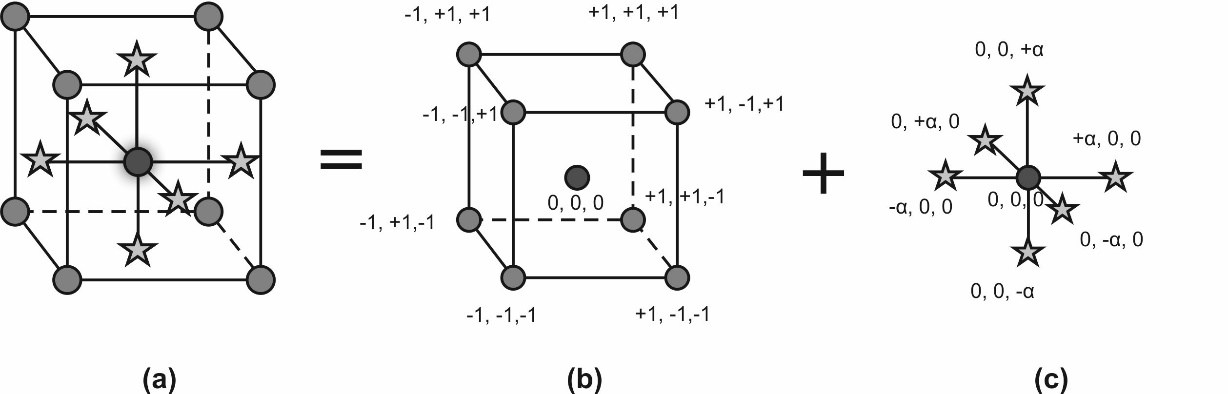Fig. 2. Face centered experimental design (a), two level factorial design and center points (b) and axial points (c) (Anderson and Whitcomb 2005)

Table 1. Factors and Corresponding Levels for the Applied Experimental Design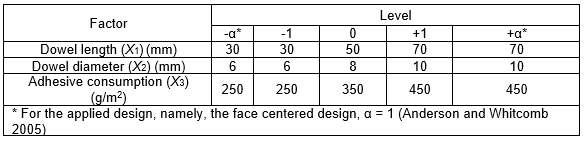The analyzed configurations are presented in Table 2. Each configuration was replicated 15 times. Therefore, a total of 450 joints were assembled and tested. Following assembly, the joints were conditioned for at least one month in the same area where the compressive and tensile tests were performed (Kasal et al. 2015). The equilibrium moisture content of heat-treated wood joints was about 5%, and the average density was equal to 618 kg/m3. Half of the joints were subjected to compression tests, and the other half were subjected to tension tests.

The mechanical testing of joints was performed on a universal testing machine (Zwick Roell Z10; Zwick GmbH & Co. KG, Ulm, Germany). The load was applied at a constant speed of 3 mm/min until a notable separation between the two parts occurred (Kuzman et al. 2015). The ultimate failure load was recorded for each analyzed dowel joint. The compression and tension tests simulated two possible situations, which could be observed when a force is applied to a typical frame structure. In the first situation, the corner joint was subjected to a compression load (Fig. 3a). In the other, the corner joint was subjected to a tension force (Fig. 3b) causing a moment tending to open the joint (Negreanu 2003; Yerlikaya and Aktaş 2012; Kasal et al. 2015).

Table 2. Configuration of Joints According to the Experimental Plan and the Mean Experimental Values with Coefficient of Variation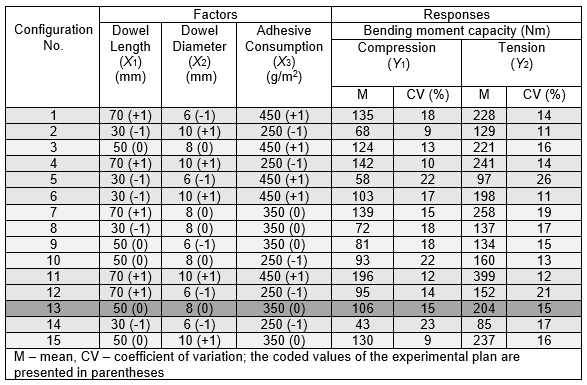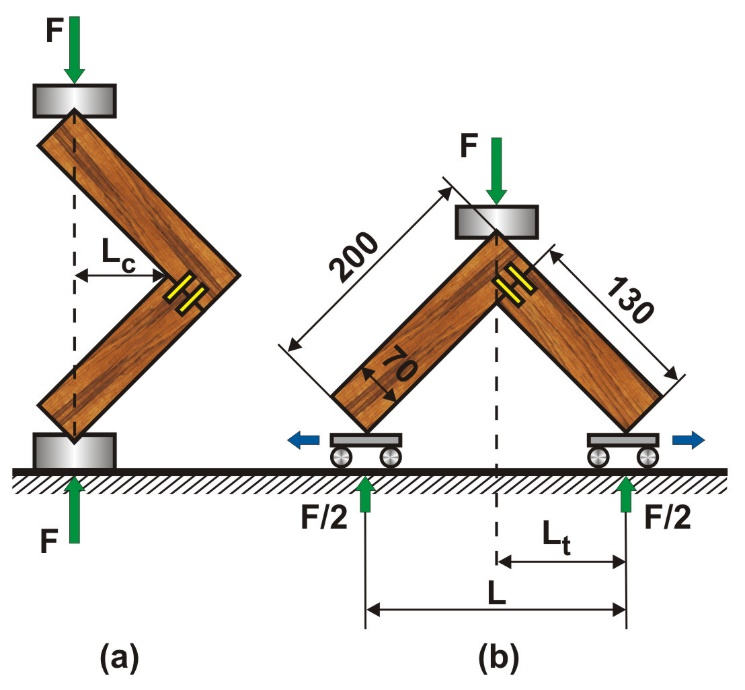Fig. 3. The loading forms of dowel joints subjected to compression (a) and tension (b) (dimensions in mm)

The bending moment capacity of the analyzed joints was calculated by means of Eq.1 for compression test and Eq. 2 for tension test (Derikvand and Eckelman 2015),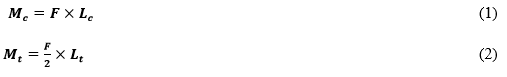where Mc is the bending moment of joints subjected to compression (Nm), Mt is the bending moment of joints loaded in tension, F is the ultimate failure load (N), Lc is compression moment arm (42 mm), and Lt is the tension moment arm (92 mm).

RESULTS AND DISCUSSION

The bending moment capacities of joints that were under a diagonal tension load were, on average, about 45% higher than those of the joints loaded in compression (Table 2). The coefficient of variation was between 9 and 23% for compression sets and between 11 and 26% for the joints loaded in tension. Therefore, the bending moment capacity in tension was more variable than that in compression.

In general, the failure modes of joints included glue-line fracture, dowel deformation, and dowel and material fracture (Fig. 4). Displacement and deformation of the dowels was also observed. Also, a separation of the parts of the joints was frequently observed when the dowel length, dowel diameter and adhesive consumption were tested at the minimum value (30 mm, 6 mm and 250 g/m2). On the other hand, when the dowel length, dowel diameter and adhesive consumption were analyzed at maximum value (70 mm, 10 mm and 450 g/m2), either material fracture or dowel fracture was observed. These failures occurred both during compression and tension testing.

Two-factor interaction (2FI) empirical models were suggested by the statistical software to predict the bending moment capacity under diagonal compression and tension loading of heat-treated wood dowel joints. The models were significant at the 1% level. The mathematical models that could predict the bending moment capacity of the joints loaded in compression (ŶMc) or tension (ŶMt) are presented in terms of both coded and actual factors (Eqs. 3 to 6):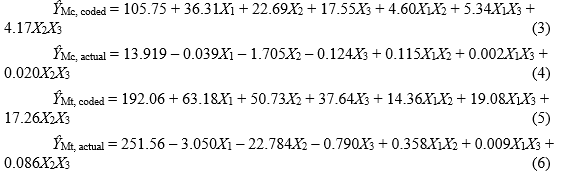The coded equations (Eqs. 3 and 5) are useful for identifying the relative impacts of the factors (Sova et al. 2016). Meanwhile, the equations in terms of actual factors (Eqs.4 and 6) can be used to make predictions about the bending moment capacity of the heat-treated dowel joints. The relation between the coded and actual values is described by the Eq. 7 (Zhu et al. 2015),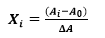(7)

where Xi is the coded value of the analyzed factor, Ais the actual value of the analyzed factor, A0 is the actual value at the center point of the factor (Table 1), ΔA is the step change.